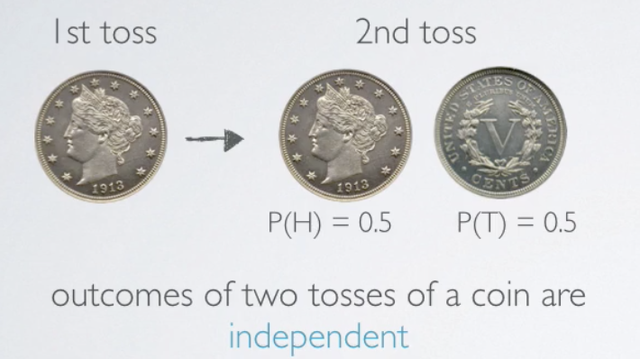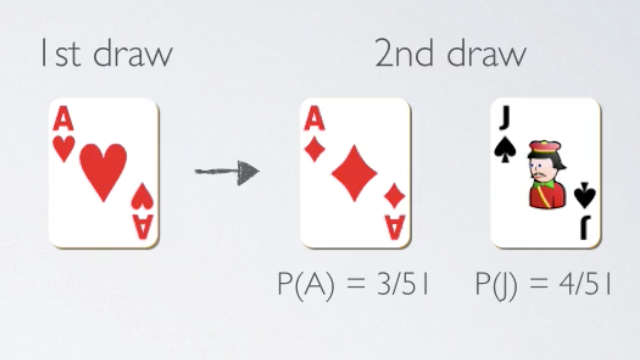### Definition of Independence

Two processes are independent if knowing the outcome of one provides no useful information about the outcome of the other.

For example 1:Knowing that the coin landed on a head on the first toss, does not provide any useful information for determining what the coin will land on in the second toss. The probability of a head or tail on the second toss is 50%, regardless the outcome of the first toss. Therefore, outcomes of two coin tosses are said to be independent.

For example 2:On the other hand, knowing that the frist card drawn from a deck is ace does provide useful information for calculating the probability of outcomes in the second draw (Drawing the cards without replacement, in other words not putting the card back into the deck). For example: the second draw to get ace is P(A) = 3/51. Therefore, outcomes of two draws from the deck of cards, without replacement are dependent.

### Checking for Independence

Based on the definition, the general rule for checking for independence between random processes is:

Rule: If the probability of an event A occuring, given that event B occured is the same as the probability of event A occuring in the first place, then events A and B are said to be independent.

`P(A | B) = P(A), then A and B are independent.`

This rule basically says that knowing B tells us nothing about A. Note: the `|` stand for given that event B occured.

### Determining dependence based on sample data

#### General procedure to determine dependence/independenceIf we observe a difference between the conditional probabilities that we calculate based on the sample, we say that these data suggest dependence. The next natural step would then be to actual conduct a hypothesis test, to see if the observed difference could have just happened due to chance or there is actually a real difference in the population.

#### Speculating based on the magnitude of probability differences

If the observed differences between the conditional probabilities is large, then there is stronger evidence that the difference is real.

For example:

Suppose that P(protects citizens | white people) = 67%, and p(protects citizens | Black people) = 24%, then there is a stronger evidence that opinion on gun ownership and race ethnicity are most likely dependent.

#### Speculating based on sample size

On the other hand, if the sample size is large, even a small difference can provide strong evidence of a real difference.

For example:

Suppose that P(protects citizens | white people) = 67%, and p(protects citizens | Black people) = 64%, then under the sample size n = 50,000 is more convinced of a real difference between the proportion of Whites and Blacks who think widespread gun ownership protects citizens. (Not n = 500)

### Product rule for independent events

Product Rule: If A and B are independent, P(A and B) = P(A) × P(B)

For example:

If you toss a coin twice, what is the probability of getting two tail in a row?

```P(two tails in a row)
= P(Tail on the 1st toss) × P(Tail on the 2nd on the 2nd toss)
= 50% × 50%
= 25%```

This rule isn't limited to just two events, it can actually be expanded to as many independent events as you need:

Expanded Product Rule: If A1, A2, ..., An are independent, P(A1 and A2 and ... An) = P(A1) × P(A2) × ... × P(An)